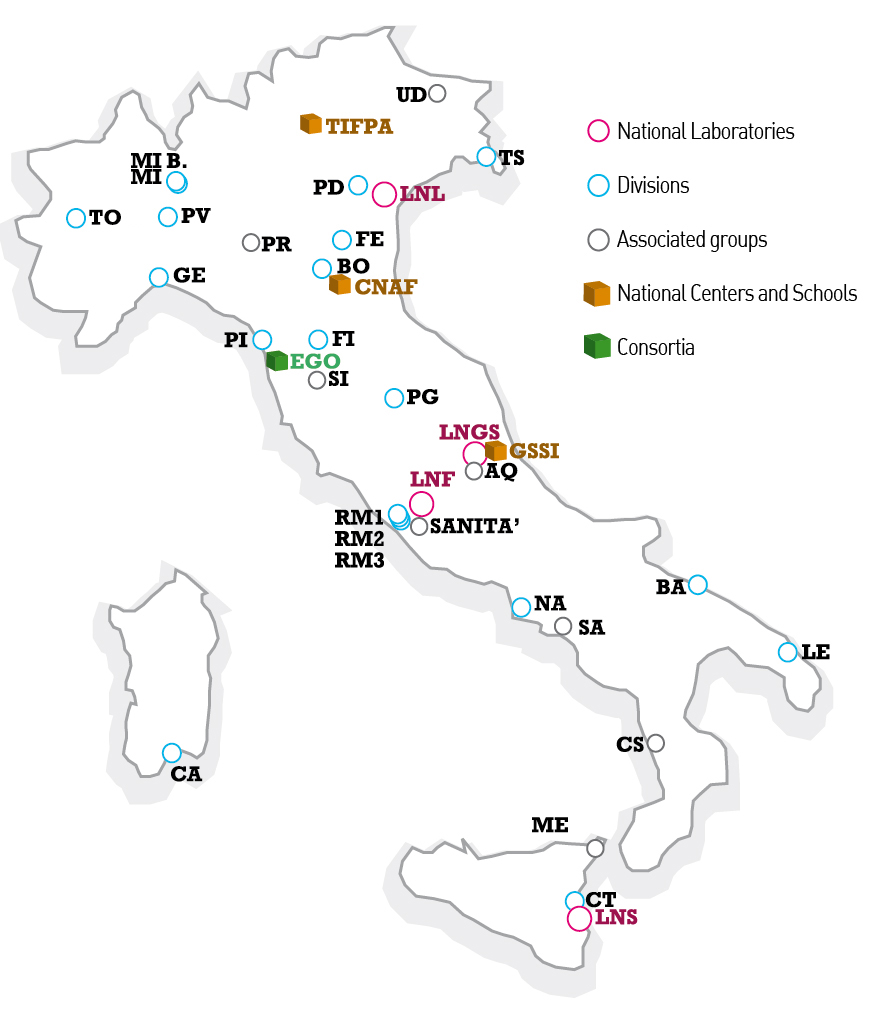# Mathematical methods of nonlinear Physics

### Abstract

Many fundamental non-linear models in Classical/Quantum Physics (Fluid, Nuclear, Condensed Matter and Plasma Physics, Optics, Gravity, Statistical, Quantum Field and String Theories) are mathematically characterized by their integrability. Integrable models, described by discrete (DDE) or continuous (PDE/ODE) differential equations, have regular stable solutions with respect to large classes of initial data, characteristic parameters and, possibly, external perturbations. The identification and the study of the main properties of integrable systems is a major area of Theoretical and Mathematical Physics.

The activities of the MMNLP research group cover the following topics in the theory of integrable systems:

1. Classification/construction of integrable systems from physical models and by algebraic/geometric methods.
2. Geometric methods in integrable systems: from symplectic to  bi-Hamiltonian geometry   with applications to Frobenius manifolds and Hitchin systems.
3. Construction of spectral methods of solution for physically relevant initial/boundary value problems of nonlinear PDEs.
4. Analytic study of extreme nonlinear wave phenomena, like the formation and dynamics of i) rogue (anomalous) waves, ii) singularities (blow up at finite time, wave breaking, shocks), iii) elliptic/hyperbolic regime transition, iv) dispersive/dissipative regularization.
5. Applications to conservation laws algebras in field/string/gravity theories and random matrix models.
6. Waves in complex classical/quantum fluids and topological field theories.
7. The ODE/IM correspondence for integrable quantum field theories.

### Map of INFN facilities### Next meeting

April, 18-19 2024